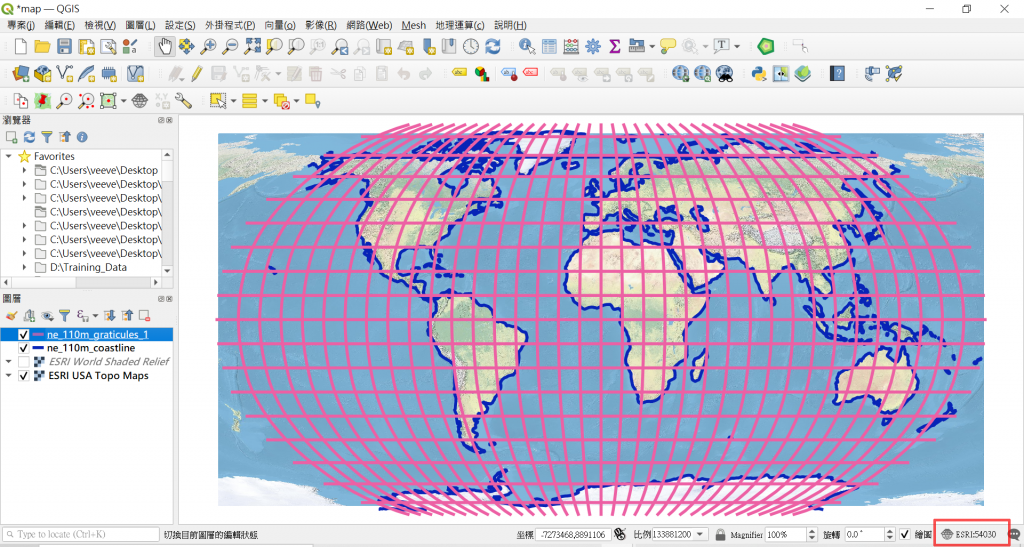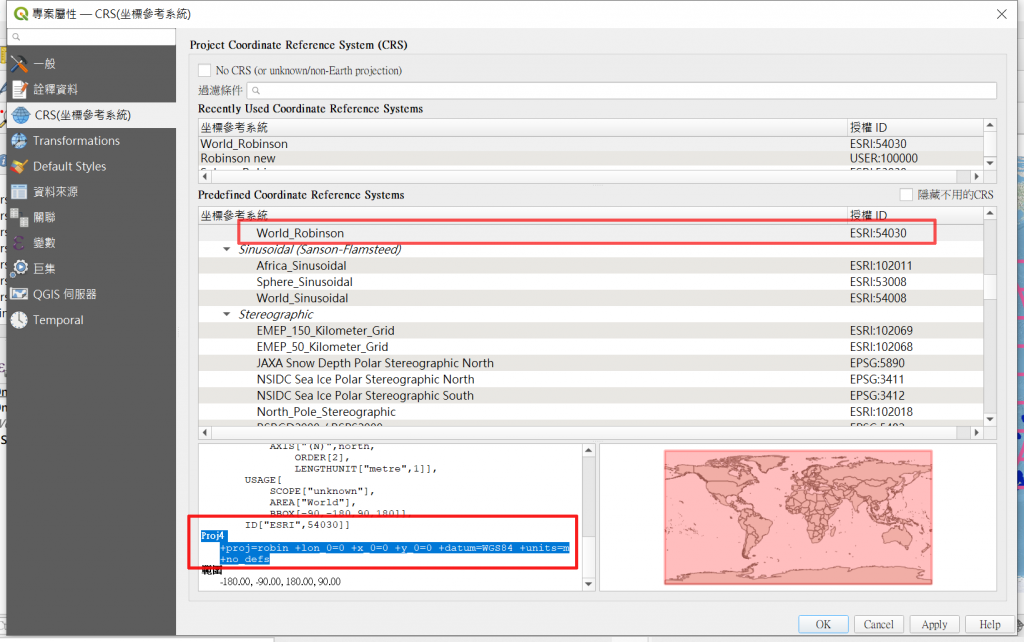# QGIS軟體改變羅賓森投影法（Robinson Projection）中央經線

1.首先將QGIS專案座標系統改為World_Robinson（ESRI：54030）2.複製World_Robinson投影的Proj4定義：

Proj4：+proj=robin +lon_0=0 +x_0=0 +y_0=0 +datum=WGS84 +units=m +no_defs3.自訂坐標參考系統定義

QGIS軟體主選單上[設定]->[Custom Projections]Proj4：+proj=robin +lon_0=-30 +x_0=0 +y_0=0 +datum=WGS84 +units=m +no_defs